# Simple harmonic motion and Hooke’s law

## Simple harmonic motion and Hooke’s law

The following text is used only for teaching, research, scholarship, educational use and informative purpose following the fair use principles.

We thank the authors of the texts and the source web site that give us the opportunity to share their knowledge

# Simple harmonic motion and Hooke’s law

## Hooke’s  Law

Hooke’s Law states that when an object is stretched the restoring force is directly proportional to the displacement, provided the elastic limit is not exceeded.

F = - k s

We represent this mathematically as follows;             F µ - s*                       Þ

The most common example of Hooke’s Law is a stretched string.
See Maths Problem 1, page 147 and Exercise 13.1, Numbers 1 and 2.

Simple Harmonic Motion (S.H.M.)*
An object is said to be moving with Simple Harmonic Motion if;

• its acceleration is directly  proportional to its distance from a fixed point in its path, and
• its acceleration is directed towards that point.

a = -w2 s

We represent this mathematically as :
• The ‘fixed point’ referred to in the definition is also called the ‘equilibrium position’.
• The key to SHM is that the acceleration and the displacement are always opposite in direction.
• This is represented by the minus sign in the equation, although mathematically the minus sign has no significance and can be ignored for calculations.

But I thought ω represented angular velocity?”*

Other examples of SHM, apart from a stretched string are:

• A prong on a vibrating tuning fork,
• A pendulum oscillating at a small angle,
• The tides.

To show that any object that obeys Hooke’s Law will also execute SHM
The aim here is to show that Hooke’s Law is a subset of S.H.M., i.e. that F µ -s can be rewritten in the form a = - w2 s.

So we start with the equation for Hooke’s Law;                    F µ -s              Þ                    F = -k s
But F =  ma                                                    Þ                 ma = -k s

Now divide both sides by m                                                              Þ                  a = -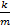s
This is equivalent to the equation for S.H.M. where the constant w2 in this case is k/m.

Relationship between Periodic Time (T) and w for an object undergoing SHM*
As with waves, the periodic time is the time taken for one complete oscillation.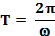Also T = 1/f, where f is the frequency.
Remember w is not angular velocity.
What is the unit of w in this particular case?

### The Simple Pendulum A simple pendulum consists of a light string with a mass at the end. For an angle of swing less than approximately 50, the motion of a Simple Pendulum can be considered to be SHM.

Periodic Time of a Simple Pendulum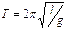Mandatory Experiment:
Investigation of the relationship between period and length for a simple pendulum, and hence calculation of g.

Leaving Cert Physics Syllabus

 Content Depth of Treatment Activities STS S.H.M. and Hooke’s law. Hooke’s law: restoring force µ displacement. F = -ks ma = -ks a =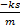= -w2s Demonstration of SHM, e.g. swinging pendulum or oscillating magnet. Appropriate calculations. Everyday examples. Systems that obey Hooke’s law e.g. simple pendulum, execute s.h.m. T =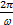INVESTIGATION OF THE RELATIONSHIP BETWEEN PERIODIC TIME AND LENGTH FOR A SIMPLE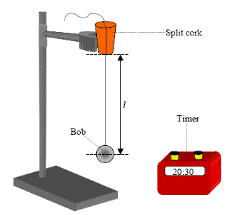PENDULUM AND HENCE CALCULATION OF g

APPARATUS
Pendulum bob, split cork, ruler, string and timer

DIAGRAM

PROCEDURE

• Place the thread of the pendulum between two halves of a cork or between two coins and clamp to a stand.
• Set the length of the thread at one metre (from the bottom of the cork or coins to the centre of the bob).

• Set the pendulum swinging through a small angle (<10°).
• Measure the time t for thirty complete oscillations.

• Divide this time t by thirty to get the periodic time T.
• Repeat for different lengths of the pendulum.

• Draw a graph of T2  against length l and use the slope to calculate a value for g ( g = 4π2/slope).

RESULTS

 l (m) time for 30 oscillations (s) time for 1 oscillation  (s) T2 (s2) g gavg

CONCLUSION
We got a value for acceleration due to gravity of 9.7 m s-2, which compares favourably with the theoretical value of 9.8
m s-2. We therefore conclude that the theory may well be correct.

SOURCES OF ERROR / PRECAUTIONS

• Measuring from the bottom of the split-cork to the centre of the bob.
• Do not cause the pendulum to swing through an angle greater than 10 degrees.
• Ensure that the pendulum swings in one plane only - avoid circular movements.
• Use a long pendulum as much as possible to minimise percentage errors associated with measuring the length and also the time.

Extra Credit
*F µ -s
Why the minus sign? Well because displacement is a vector, it has a direction associated with it, and this direction is always opposite to the direction of the restoring force, even though as one quantity gets bigger so does the other.

## Basically what’s going on is that an object has its natural resting point, and if, when it gets disturbed from this point it tries to return but actually overshoots the mark and has to return again and again, then the object is executing SHM.

In our study of Newton’s Laws of Motion we consider the motion of a body when subject to a constant force or forces. As a result we can calculate the object’s velocity or position at any time.

However there are many instances when a moving object is subject to a changing force – can we still calculate future position and velocity? Well, we can if we can quantify the force, i.e. if we know how the force is changing. One example of an object experiencing a changing force is a stretched spring. We say that the spring is undergoing Simple Harmonic Motion (SHM).

What happens acceleration in Simple Harmonic Motion?
Recap

• In linear motion, acceleration is always constant in direction and magnitude
• In SHM, acceleration by its nature is not constant in either direction or magnitude.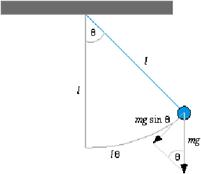Let’s take another look at this.
Consider a Simple Pendulum*

 displacement velocity acceleration Equiplibrium position 0 max 0 Extreme position max 0 max

The aspect which most people find confusing here is that at the extremities the velocity is zero, while the acceleration is a maximum, whereas at the equilibrium position the opposite is the case.  How can this be?
You must think in terms of forces
Personally, I can’t fathom why textbooks don’t explain this by referring to forces; after all, the only way you can have acceleration is if you have a force causing it.
Acceleration is directly proportional to the force causing it, so if force is a maximum acceleration must be also, and vice versa. Now let’s look at the diagram in terms of forces.
At the equilibrium point the restoring force on the mass is zero  – it’s not being pulled to the left or the right, therefore the acceleration must be zero, whereas at the extremities the force pulling the mass back in is a maximum, therefore the corresponding acceleration must also be also be a maximum.

The other point which might cause confusion is velocity being zero at the extreme positions, but remember we came across this before; when an object is thrown up its instantaneous velocity is zero at the very top, (even though its acceleration is not zero).

* “But I thought ω represented angular velocity?”
Well spotted. ω is the same symbol as was used in the last chapter (Circular Motion) to represent angular velocity, however  it does not represent angular velocity here. It’s not just coincidence that ω turns up in both chapters; they are connected, but the relationship is a little complicated.

In the last chapter we made use of the formula v = distance/time and applied it to a satellite undergoing a full cycle where v = 2πr /T . We also had v = rω, so we can re-write the previous equation as rω = 2π r/T. Cancel r’s to get ω = 2π /T.
Cross-multiply to get T = 2π/ω.
Hmmm . . . - what does a stretched string have to do with circular motion?

Exam Questions

• 

State Hooke’s law.

• 

A mass at the end of a spring is an example of a system that obeys Hooke’s Law.
Give two other examples of systems that obey this law.

• 
• The equation F = – ks, where k is a constant, is an expression for a law that governs the motion of a body.

Name this law and give a statement of it.

• Give the name for this type of motion and describe the motion.
• 
• When a sphere of mass 500 g is attached to a spring of length 300 mm, the length of the spring increases to 330 mm. Calculate the spring constant.
• The sphere is then pulled down until the spring’s length has increased to 350 mm and is then released.

Describe the motion of the sphere when it is released.

• What is the maximum acceleration of the sphere?
• 
• When a small sphere of mass 300 g is attached to a spring of length 200 mm, its length increases to 285 mm.

Calculate its spring constant.

• The sphere is pulled down until the length of the spring is 310 mm.

The sphere is then released and oscillates about a fixed point.
Derive the relationship between the acceleration of the sphere and its displacement from the fixed point.

• Why does the sphere oscillate with simple harmonic motion?
• Calculate the period of oscillation of the sphere.
• Calculate the maximum acceleration of the sphere.
• Calculate the length of the spring when the acceleration of the sphere is zero.
• 
• The springs of a mountain bike are compressed vertically by 5 mm when a cyclist of mass 60 kg sits on it.

When the cyclist rides the bike over a bump on a track, the frame of the bike and the cyclist oscillate up and down.
Using the formula F = – ks, calculate the value of k, the constant for the springs of the bike.

• The total mass of the frame of the bike and the cyclist is 80 kg.

Calculate the period of oscillation of the cyclist.

• Calculate the number of oscillations of the cyclist per second.

Section A questions

• 

A student investigated the relationship between the period and the length of a simple pendulum. The student measured the length l of the pendulum.
The pendulum was then allowed to swing through a small angle and the time t for 30 oscillations was measured.
This procedure was repeated for different values of the length of the pendulum.
The student recorded the following data:

 l /cm 40 50 60 70 80 90 100 t /s 38.4 42.6 47.4 51.6 54.6 57.9 60
• Why did the student measure the time for 30 oscillations instead of measuring the time for one?
• How did the student ensure that the length of the pendulum remained constant when the pendulum was swinging?
• Using the recorded data draw a suitable graph to show the relationship between the period and the length of a simple pendulum.
• What is this relationship?
• Use your graph to calculate the acceleration due to gravity.

• 

In investigating the relationship between the period and the length of a simple pendulum, a pendulum was set up so that it could swing freely about a fixed point.
The length l of the pendulum and the time t taken for 25 oscillations were recorded.
This procedure was repeated for different values of the length.
The table shows the recorded data.

 l/cm 40 50 60 70 80 90 100 t/s 31.3 35.4 39.1 43 45.5 48.2 50.1

The pendulum used consisted of a small heavy bob attached to a length of inextensible string.

• Explain why a small heavy bob was used.
• Explain why the string was inextensible.
• Describe how the pendulum was set up so that it swung freely about a fixed point.
• Give one other precaution taken when allowing the pendulum to swing.
• Draw a suitable graph to investigate the relationship between the period of the simple pendulum and its length.
• What is this relationship?

Exam Solutions

• For a stretched string the restoring force is proportional to the extension.

A stretched spring obeys Hooke’s law.

• Stretched elastic, pendulum, oscillating magnet, springs of car, vibrating tuning fork, object bobbing in water waves, ball in saucer, etc.

•
• Hooke’s Law states that when an object is stretched the restoring force is directly proportional to the displacement, provided the elastic limit is not exceeded.
• Simple harmonic motion; an object is said to be moving with Simple Harmonic Motion if its acceleration is directly proportional to its distance from a fixed point in its path, and its acceleration is directed towards that point.
•
• When the mass of 500 g is attached the new force down = mg = (0.5)(g).

Because the spring is in equiblibrium this must be equal to the force up (which is the restoring force).
Hooke’s law in symbols: F = k x
Þ (0.5)(g) = kx     Þ k = F/x = 0.5g/0.030                      Þ k = 163.3 N m-1

• It executes simple harmonic motion because the displacement is proportional to t he acceleration.
• F = ma = kx

Þ a = kx/m = (163.3)(0.02)/(0.5) = 6.532 m s-2
OR
a = w2 x Þ            w2 = k/m = 163.3/0.5 Þ a = 6.532 m s-2

•
• F = ks

mg  = ks
(0.30)(9.8) = (k)(0.085)
k = 34.6 N m-1

• F = - ks      Þ        ma = - ks         Þ        a = - (k/m)s      Þ        a α -s Þ        a = - k s
• Its acceleration is proportional to its displacement from a fixed point.
• From above: ω2 = k/m  Þ  ω2 = 34.6 / 0.3           Þω = 10.7 ÞT = 2π/ω  = 2π/10.7 = 0.58 ≈ 0.6          Þ T = 0.6 s
• This occurs when s is a maximum, i.e. when s = amplitude = 0.310 – 0.285 = 0.025 m.

a = -ω2s     Þ        a = - (10.7)2 (0.025)    Þ        a = (-) 2.89 m s-2

• This occurs at the fixed point when l = 0.285 m
•
• F = – ks     Þ  mg = – ks   Þ 60 × 9.8 = -k (.005)            Þ  588 = -k (.005)      Þ k  = 1.2 × 105  N m-1
• k/m = ω2

ω  = 38 s-1
T = 2π/ω = 0.16 s

• f = 1/T  ≈ 6

Solutions to Section A questions

•
• To reduce percentage error in measuring the periodic time.
• Use an inextensible string, string suspended at fixed point (e.g. split cork or two coins)

•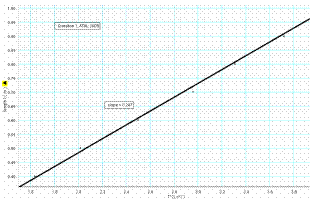See graph

 l /cm 40.0 50.0 60.0 70.0 80.0 90.0 100.0 t /s 38.4 42.6 47.4 51.6 54.6 57.9 60.0 Time for 1 swing/ s t2/ s2 l /m 0.4 0.5 0.6 0.7 0.8 0.9 1.0

• t2 is proportional to l
• Slope = 0.25 (ms–2) [range: 0.24 – 0.25 m s–2]

g = 9.72 m s–2 [range: 9.4 – 9.9 m s–2]

•
• To reduce air resistance and to keep the string taut
• So that length remains constant because length would be another variable.
• The string was placed between two coins (or a split cork).
• Make sure that there are no draughts / make sure it oscillates in one plane only.
• Draw a suitable graph
 time for 25 swings /s 31.3 35.4 39.1 43 45.5 48.2 time for 1 swing/s 1.25 1.42 1.56 1.72 1.82 1.93 t2/s2 1.57 2.01 2.45 2.96 3.31 3.72 l/m 0.4 0.5 0.6 0.7 0.8 0.9

Values of t divided by 25 to get T
Axes correctly labelled  T2 vs. l
At least six points plotted correctly
Straight line drawn

• T2 is proportional to l
• The graph resulted in a straight line through the origin

Source : http://www.thephysicsteacher.ie/LC%20Physics/Student%20Notes/13.%20Hookes%20Law%20and%20SHM.doc

Author : not indicated on the source document of the above text

If you are the author of the text above and you not agree to share your knowledge for teaching, research, scholarship (for fair use as indicated in the United States copyrigh low) please send us an e-mail and we will remove your text quickly.

### Simple harmonic motion and Hooke’s law

#### Simple harmonic motion and Hooke’s law

Main page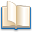Alimentation - nutritionAnimalsArchitectureArtAstrology, esoteric, parapsychology and fantasyAstronomyBiology and geneticsBotany and agricultureChemistryCourses, training, guides, handbooks and tipsCulture General and actualityDermatology, health and wellnessDictionaries and glossariesEconomics and financeEducation and pediatricsEngineering and technologyEnglish grammar and anthologyFashion and show businessFitnessFood, recipes and drinkGames, play spaceGeography, geology and environmentHistoryInformatics and computer worldJobs worldLanguagesLeisure and DIY do it yourselfLessons for studentsLiterature, biographies and journalismMathematics, geometry and statisticalMedicineMusicNotes and concept mapsPhilosophyPhotography and images - picturesPhysicsPhysiologyPsychology and psychoanalysisQuiz questions and answersReligionsRights law and political scienceScienceSociology and cultural anthropologySportSummaries and reviewsTelevision, film - movies and comicsTheater and communicationsTheses, themes and dissertationsTravel and tourist guidesUseful documents and tablesUtility, calculators and convertersWeather and meteorology

#### Simple harmonic motion and Hooke’s law

This is the right place where find the answers to your questions like :

Who ? What ? When ? Where ? Why ? Which ? How ? What does Simple harmonic motion and Hooke’s law mean ? Which is the meaning of Simple harmonic motion and Hooke’s law?

##### Simple harmonic motion and Hooke’s law physics notes

Alanpedia.com from 1998 year by year new sites and innovations